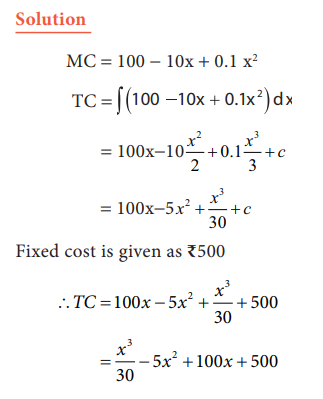Home | | Economics 11th std | Integral Calculus

# Integral Calculus

Differential calculus measures the rate of change of functions.

Integral Calculus

## 1. Integration

Differential calculus measures the rate of change of functions. In Economics it is also necessary to reverse the process of differentiation and find the function F(x) whose rate of change has been given. This is called integration. The function F(x) is termed an integral or anti- derivative of the function f(x).

The integral of a function f(x) is expressed mathematically asHere the left hand side of the equation is read “the integral of f(x) with respect to x” The symbol ∫ is an integral sign, f(x) is integrand, C is the constant of integration, and F(x)+c is an indefinite integral. It is so called because, as a function of x, which is here unspecified, it can assume many values.

## 2. Meaning

If the differential coefficient of F (x) with respect to x is f(x), then an integral of f(x)

with respect to x is F(x) . It is a reverse process of differentiation. In symbols:Following points need to be remembered:

a. ∫  is used to denote the process of integration. In fact, this symbol is an elongated ‘S’ denoting sum.

b. The differential symbol ‘dx’ is written by the side of the function to be integrated.

c. ∫ f  x dx = F(x)+C, C is the integral constant

∫ f x dx means, integration of f(x) with respect to x.

## 3. Basic Rule of Integration## 4. Application of Integration

Example 12.26

Let the marginal cost function of a firm be 100-10x+0.1x2 where x is the output. Obtain the total cost function of the firm under the assumption that its fixed cost is  Rs.500.Example 12.27

The marginal cost function for producing x units is y = 23 + 16x - 3x2 and the total cost for producing zero unit is  Rs.40. Obtain the total cost function and the average cost function.

Solution:

Given the marginal cost function y = 23 + 16x - 3x2 ; C = 40

Rs.40 is the fixed cost.

We know that

Total Cost function = ∫ (Marginal cost function) dx+C## 5. Consumer’s Surplus

This theory was developed by the Alfred Marshall. The demand function P(x) reveals the relationship between the quantities that the people would buy at given price. It can be expressed as

P = f (x)

Consumer surplus is the difference between the price one is willing to pay and the price that is actually paid.

It is represented in the following diagram.Mathematically, the consumer’s surplus (CS) can be defined asExample:12.28

If the demand function is P = 35 - 2x - x2 and the demand x0 is 3, what will be the consumer’s surplus?

Solution

Given demand function,

P = 35 - 2x - x2

for x = 3

=   35 - 2(3) -32

=35 - 6 - 9

P = 20

Therefore,

CS = (Area of the curve below the demand curve from 0 to 3) - Area of the rectangle (20 x 3 = 60)## 6. Producer’s surplus

Example 12.29

Given the demand function Pd = 25 - Q2 and the supply function Ps = 2Q + 1.

Assuming pure competition, find (a) consumers surplus and (b) producers surplus. (Pd =Demand price; Ps = Supply price)

Solution:

For market equilibrium, Pd = Ps

25-Q2 = 2Q+1

= -25 + Q2 + 2Q+1

0 = -24 + Q2 + 2Q

Q2 + 2Q - 24 = 0

Q2 + 6Q - 4Q - 24 = 0

Q(Q + 6) -4(Q + 6) = 0

(Q + 6)(Q- 4) = 0

So, Q = 4 or Q = -6. Since Q cannot be equal to -6,

Q = 4=36 - (16 + 4) = 16

Think and Do

Find your consumption of petrol for an additional unit of kilometer travelled